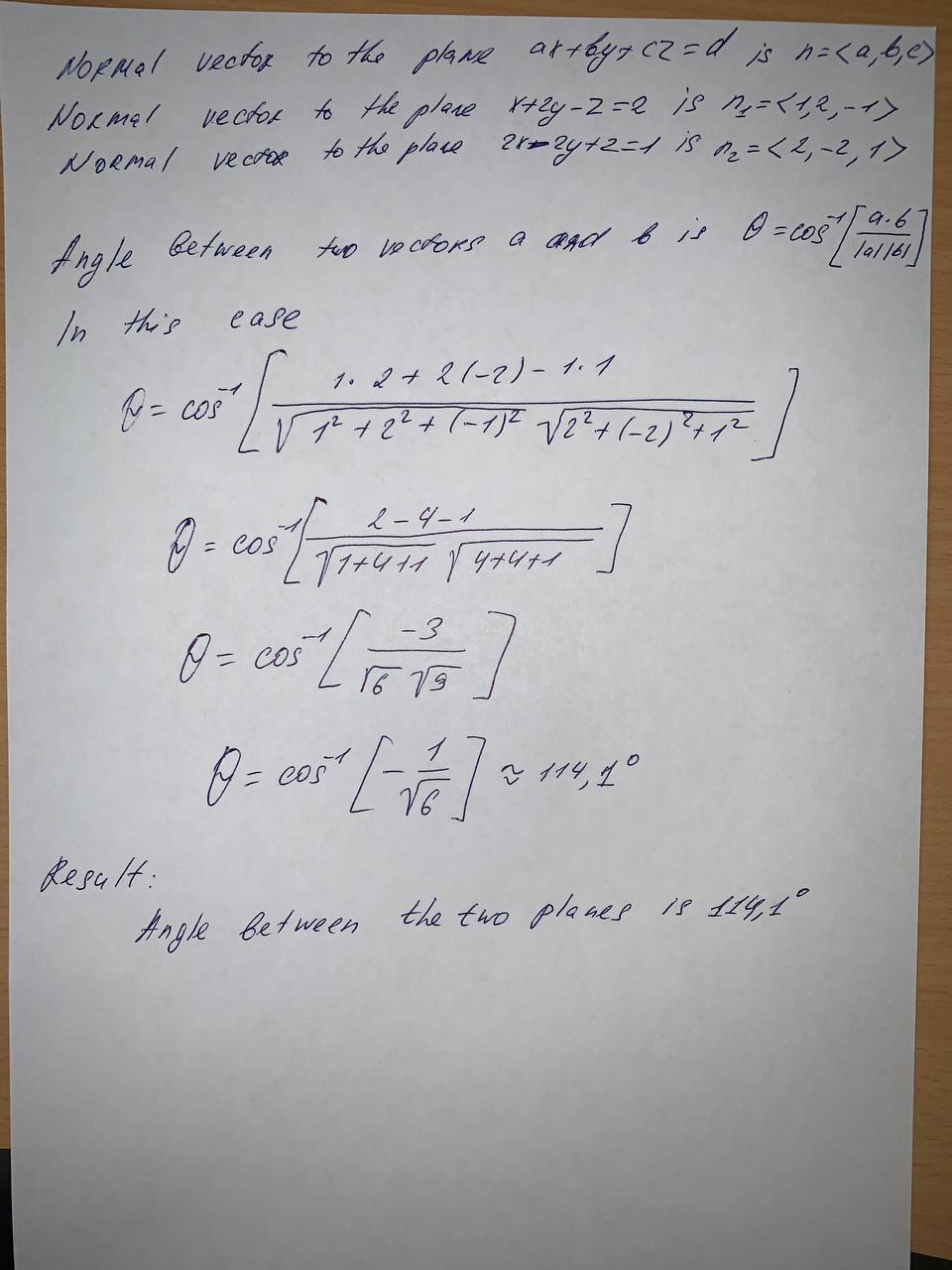# Question# Determine whether the planes are parallel, perpendicular, or neither. If neither, find the angle between them. (Round to onedecimal place.)x+2y-z=2,2x-2y+z=1

Vectors and spaces
ANSWEREDDetermine whether the planes are parallel, perpendicular, or neither. If neither, find the angle between them. (Round to one decimal place.)
$$x+2y-z=2,$$
$$2x-2y+z=1$$